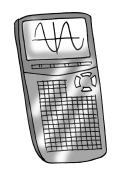### Home > CCA2 > Chapter 6 > Lesson 6.2.4 > Problem6-140

6-140.

Using your calculator, solve the equations below. Round answers to the nearest $0.001$.

Undo powers with fractional exponents. If $x$ is not in the exponent, you should not use logs.1. $x^6=125$

1. $x^{3.8}=240$

1. $x^{−4}=100$

1. $(x+2)^3=65$

$x=2.021$

1. $4(x−2)^{12.5}=2486$

Divide by $4$ first.

$x=3.673$